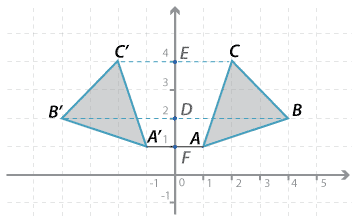### Reflection in the y-axisDetailed description

The point $$A$$ in the diagram above has been reflected in the y-axis. To reflect $$A$$, a line has been drawn at right angles to the axis of reflection (the $$y$$-axis in this case) and a ruler or compass used to mark $$A^\prime$$ the same distance from the axis of reflection, but on the other side. Similarly for $$B$$ and $$C$$.

Using coordinates we can describe the reflection of the vertices:

$$A(1,1) \rightarrow A^\prime (−1,1); B(4,2) \rightarrow B^\prime (−4,2); C(2,4) \rightarrow C^\prime (−2,4)$$